﻿ 基于VMD与BP神经网络的短期电价预测

# 基于VMD与BP神经网络的短期电价预测Short-Term Electricity Price Forecasting Based on VMD and BP Neural Network

Abstract: Electricity price is one of the most important indexes in electricity market transactions. Electricity market participants adjust their investment strategies to participate in power transactions based on forecast prices. To improve the accuracy of short-term price forecasting in electricity market, a short-term electricity price forecasting method based on VMD and BP neural network is proposed in the paper. The proposed method has the following three steps: 1) VMD is used to decompose historical electricity price data into different modal functions. 2) BP neural network is applied to forecast the results of decomposition. 3) The forecasting results are reconstructed to get the short-term electricity price prediction results. Finally, this method is applied to the PJM electricity market in the United States, and the superiority of using VMD as a preconditioning algorithm for historical electricity price signals is verified. Simulation results shows that the forecasting result can fit the actual data well and have a higher prediction accuracy comparing with the forecasting results based on BP neural network only.

1. 引言

2. 原理和方法

2.1. 变分模态分解

VMD算法通过搜索约束变分模型最优解来实现信号的自适应分解   ，迭代求解变分模型，最终可以根据被分解信号的频域特性完成信号频带的自适应分解。对于原始信号f，其对应的约束变分模型表达式如公式(1)所示。

$\left\{\begin{array}{l}\underset{\left\{{\mu }_{k}\right\},\left\{{\omega }_{k}\right\}}{\mathrm{min}}\left\{{\underset{k\text{=}1}{\overset{K}{\sum }}‖{\partial }_{t}\left[\left(\delta \left(t\right)+\frac{j}{\pi t}\right){u}_{k}\left(t\right)\right]{\text{e}}^{-j{\omega }_{k}t}‖}_{2}^{2}\right\}\hfill \\ s.t.\text{\hspace{0.17em}}\underset{k\text{=}1}{\overset{K}{\sum }}{u}_{k}=f\hfill \end{array}$ (1)

$L\left(\left\{{u}_{k}\right\},\left\{{\omega }_{k}\right\},\left\{\lambda \right\}\right)=\alpha {\underset{k=1}{\overset{K}{\sum }}‖{\partial }_{t}\left[\left(\delta \left(t\right)+\frac{j}{\pi t}\right){u}_{k}\left(t\right)\right]{\text{e}}^{-j{\omega }_{k}t}‖}_{2}^{2}+{‖f\left(t\right)-\underset{k=1}{\overset{K}{\sum }}{u}_{k}\left(t\right)‖}_{2}^{2}+〈\lambda \left(t\right),f\left(t\right)-\underset{k=1}{\overset{K}{\sum }}{u}_{k}\left(t\right)〉$ (2)

${u}_{k}^{n+1}=\underset{{u}_{k}\in X}{\mathrm{arg}\mathrm{min}}\left\{\alpha {‖{\partial }_{t}\left[\left(\delta \left(t\right)+\frac{j}{\pi t}\right)\ast {u}_{k}\left(t\right)\right]{\text{e}}^{-j{\omega }_{k}t}‖}_{2}^{2}+{‖f\left(t\right)-\underset{i\ne k}{\overset{}{\sum }}{u}_{i}\left(t\right)+\frac{\lambda \left(t\right)}{2}‖}_{2}^{2}\right\}$ (3)

${\stackrel{^}{u}}_{k}^{n+1}\left(\omega \right)=\frac{\stackrel{^}{f}\left(\omega \right)-\underset{ik}{\sum }{\stackrel{^}{u}}_{i}^{n}\left(\omega \right)+\stackrel{^}{\lambda }\left(\omega \right)/2}{1+2\alpha {\left(\omega -{\omega }_{k}\right)}^{2}}$ (4)

${\omega }_{k}^{n+1}=\underset{{\omega }_{k}}{\mathrm{arg}\mathrm{min}}\left\{{‖{\partial }_{t}\left[\left(\delta \left(t\right)+\frac{j}{\pi t}\right)\ast {u}_{k}\left(t\right)\right]{\text{e}}^{-j{\omega }_{k}t}‖}_{2}^{2}\right\}$ (5)

${\omega }_{k}^{n+1}=\frac{{\int }_{0}^{\infty }\omega {|{\stackrel{^}{u}}_{k}^{n+1}\left(\omega \right)|}^{2}\text{d}\omega }{{\int }_{0}^{\infty }{|{\stackrel{^}{u}}_{k}^{n+1}\left(\omega \right)|}^{2}\text{d}\omega }$ (6)

$|{\stackrel{^}{u}}_{k}\left(\omega \right)|$ 进行傅里叶逆变换，取实部即可得到时域模态分量 $\left\{{u}_{k}\left(t\right)\right\}$ 。Lagrange算子 $\lambda$ 按式(7)更新。

${\stackrel{^}{\lambda }}^{n+1}\left(\omega \right)={\stackrel{^}{\lambda }}^{n}\left(\omega \right)+\tau \left(\stackrel{^}{f}\left(\omega \right)-\underset{k}{\sum }{\stackrel{^}{u}}_{k}^{n+1}\left(\omega \right)\right)$ (7)

VMD算法的交替迭代过程如图1所示。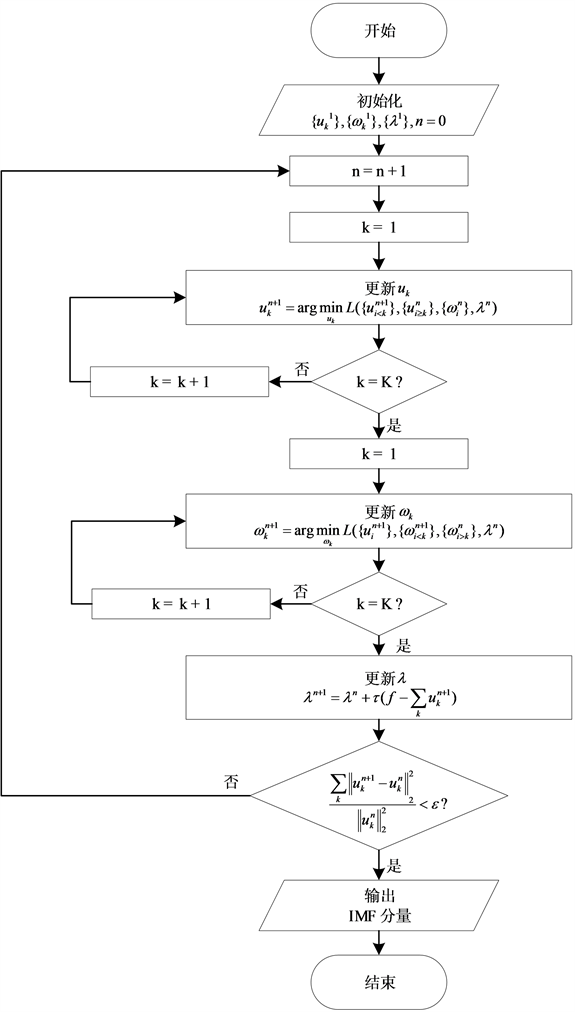Figure 1. VMD flowchart

2.2. BP神经网络

BP神经网络是一种多层前馈神经网络，其结构主要由输入层、隐层和输出层三部分组成，BP网络的学习过程由前向计算和误差反向传播两部分组成  。假设在一个BP神经网络中有N个输入、K个隐层和M个输出。如果BP神经网络的输入是Xpn，期望输出是Tpm，隐藏层输出是Y1pk，输出层输出是Y2pm，输入层和隐藏层的连接权重是W1nk，隐层和输出层的连接权重是W2km，允许误差是ε，则BP神经网络的结构图如图2所示。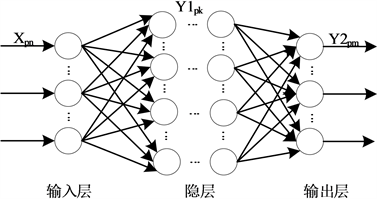Figure 2. The structure diagram of the BP neural network

$\begin{array}{l}Y{1}_{pk}\left(i\right)=f\left(\underset{n=1}{\overset{N}{\sum }}W{1}_{nk}\left(i\right){X}_{pn}\right)\\ Y{2}_{pm}\left(i\right)=f\left(\underset{k=1}{\overset{K}{\sum }}W{2}_{km}\left(i\right)Y{1}_{pk}\left(i\right)\right)\end{array}$ (8)

$\begin{array}{l}W{1}_{nk}\left(i+1\right)=W{1}_{nk}\left(i\right)+\Delta W{1}_{nk}\left(i+1\right)\\ W{2}_{km}\left(i+1\right)=W{2}_{km}\left(i\right)+\Delta W{2}_{km}\left(i+1\right)\end{array}$ (9)

2.3. 短期电价预测方法

1) 利用VMD算法将历史电价数据分解为K个模态函数。

2) 将得到的K个模态函数分别作为BP神经网络输入层的输入量，经BP神经网络计算，BP神经网络输出层输出每个模态函数的预测值。

3) 将各模态函数的预测值叠加，得到短期电价的预测值。Figure 3. The flowchart of the short-term electricity price forecasting

3. 算例分析

3.1. 历史电价信号模态分解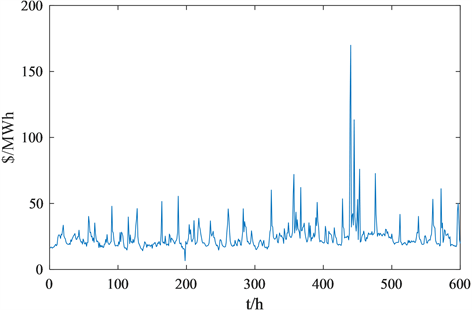Figure 4. Historical electricity price data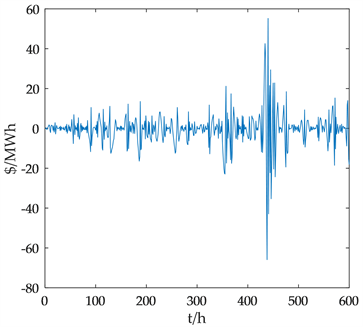(a) IMF1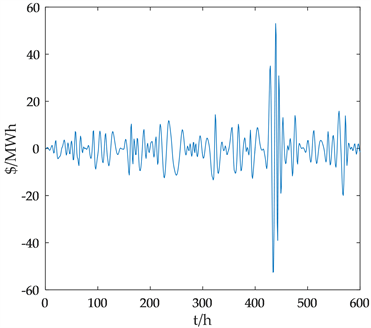(b) IMF2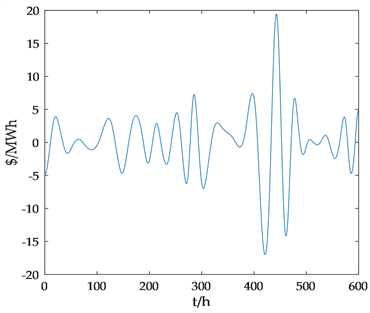(c) IMF3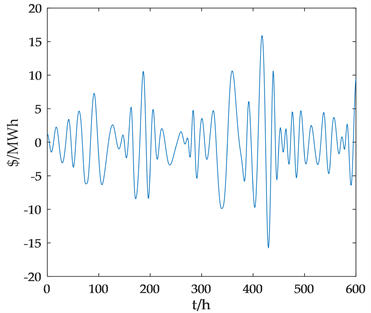(d) IMF4(e) IMF5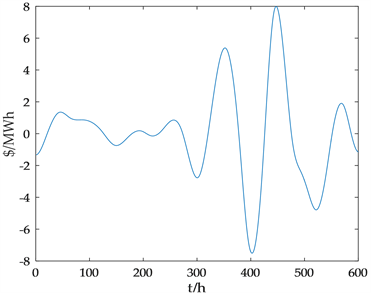(f) IMF6

Figure 5. Modal functions of EMD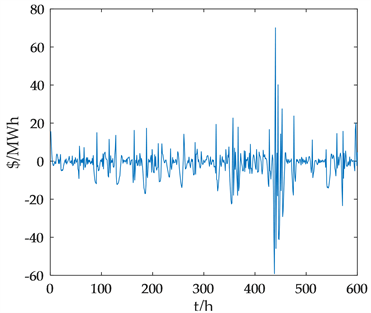(a) IMF1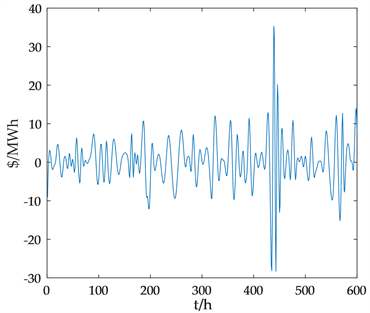(b) IMF2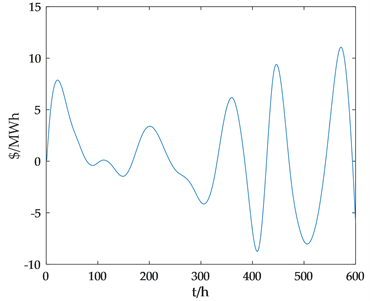(c) IMF3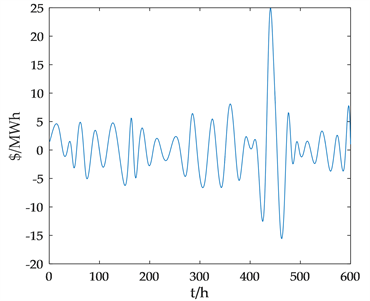(d) IMF4(e) IMF5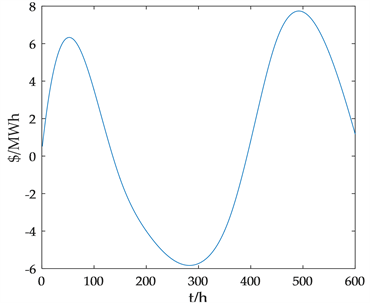(f) IMF6

Figure 6. Modal functions of LMD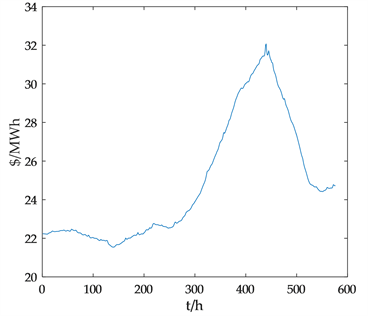(a) IMF1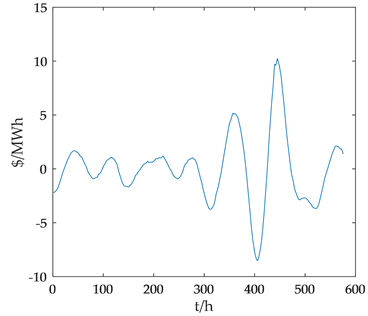(b) IMF2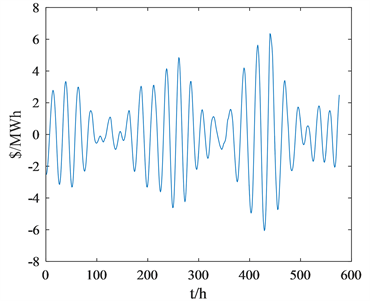(c) IMF3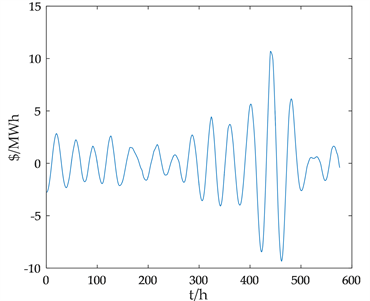(d) IMF4(e) IMF5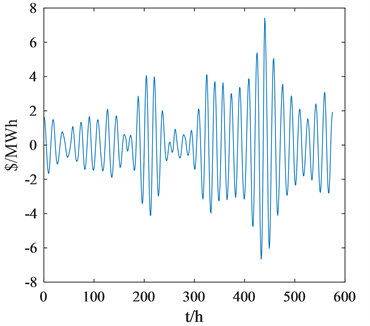(f) IMF6

Figure 7. Modal functions of VMD

3.2. 模态函数预测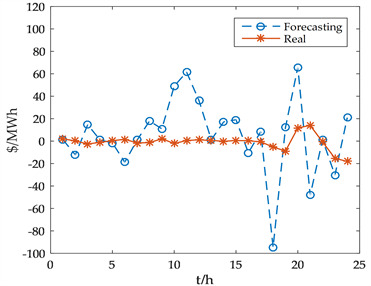(a) F_IMF1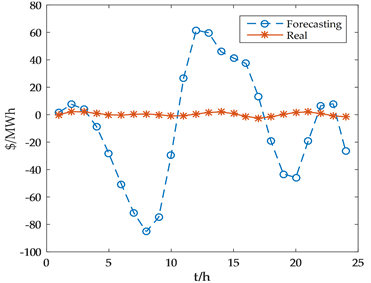(b) F_IMF2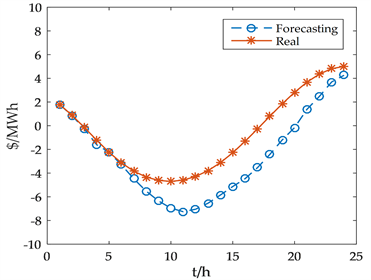(c) F_IMF3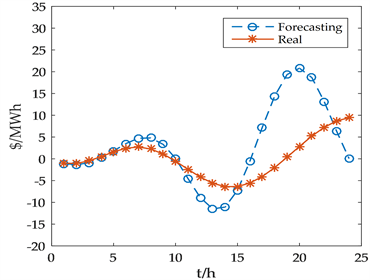(d) F_IMF4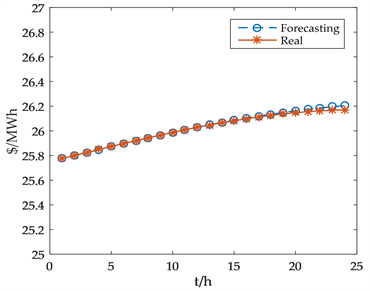(e) F_IMF5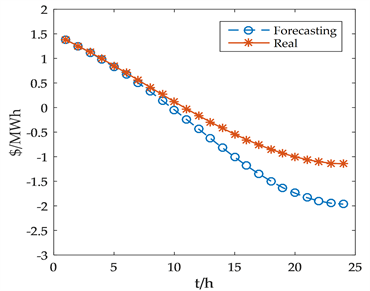(f) F_IMF6

Figure 8. Modal functions forecasting of EMD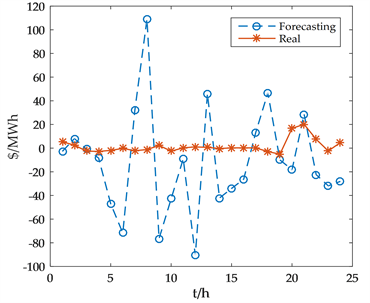(a) F_IMF1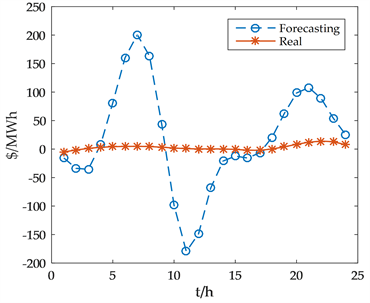(b) F_IMF2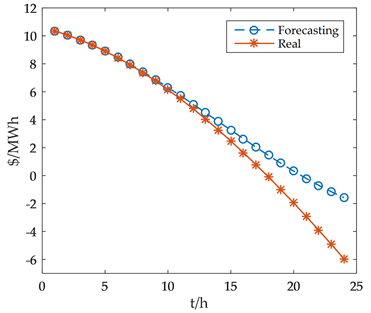(c) F_IMF3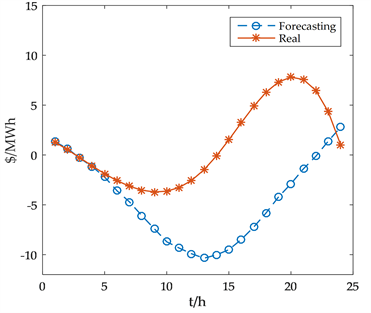(d) F_IMF4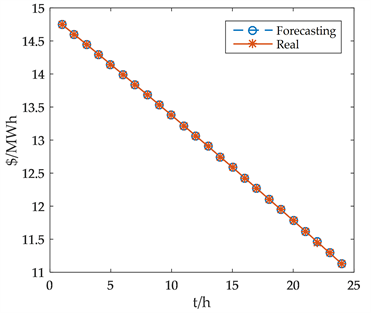(e) F_IMF5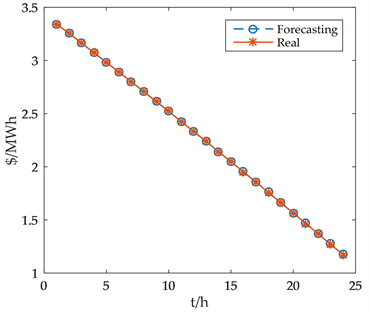(f) F_IMF6

Figure 9. Modal functions forecasting of LMD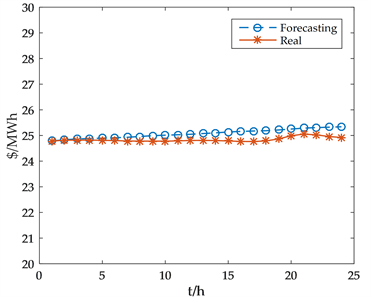(a) F_IMF1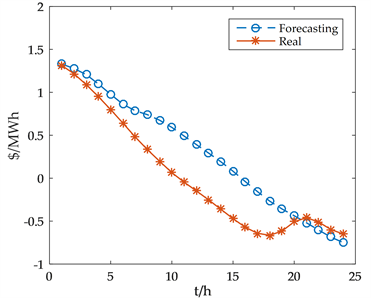(b) F_IMF2(c) F_IMF3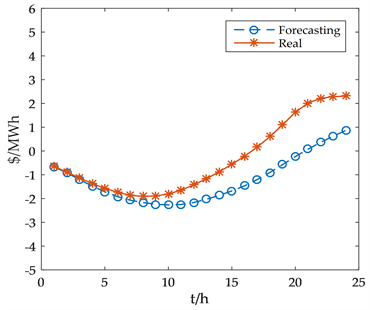(d) F_IMF4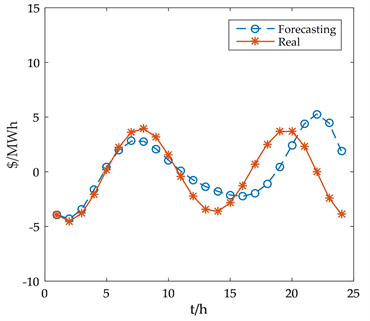(e) F_IMF5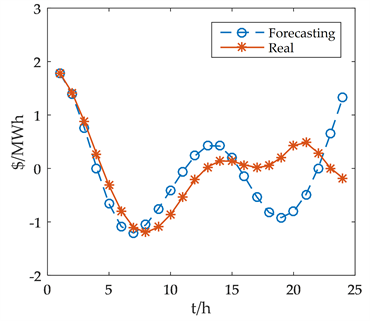(f) F_IMF6

Figure 10. Modal functions forecasting of VMD

3.3. 短期电价预测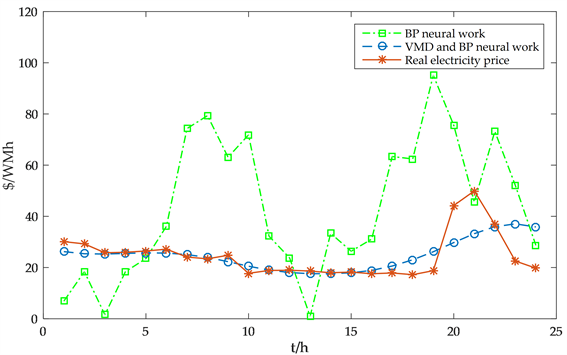Figure 11. Comparison of forecasting results

1) 影响短期电价的因素很多，不同的因素对短期电价有不同的影响。因此，仅使用BP神经网络进行电价预测会产生误差。

2) 由于电价的采样时间间隔为一小时，历史电价数据波动较大，历史电价数据中存在许多尖点，导致直接预测结果不准确。

1) 通过对历史电价进行VMD分解，可以得到多个模态函数，并且通过分解得到的每个模态函数表示不同因素对电价的影响。因此，利用各模态函数对电价进行预测，可以提高预测精度。

2) VMD分解结果既保持了原电价的趋势，又具有相对平滑的波形，克服了数据波动大的问题。

4. 结论

 Zhang, X. and Wang, X.F. (2006) Review of the Short-Term Electricity Price Forecasting. Automation of Electric Power Systems, 30, 92-101.

 Catalão, J.P.S. (2017) Electric Power Systems Advanced Forecasting Techniques and Optimal Generation Scheduling. CRC Press, Boca Raton.

 Keles, D., Scelle, J., Paraschiv, F., et al. (2016) Extended Forecast Methods for Day-Ahead Electricity Spot Prices Applying Artificial Neural Networks. Applied Energy, 162, 218-230.
https://doi.org/10.1016/j.apenergy.2015.09.087

 Hernandez, L., Baladrón, C., Aguiar, J.M., et al. (2013) Short-Term Load Forecasting for Microgrids Based on Artificial Neural Networks. Energies, 6, 1385-1408.
https://doi.org/10.3390/en6031385

 周黎英, 赵国树. 人工神经网络在电价预测中的应用[J]. 水电能源科学, 2010, 28(6): 153-156.

 Szkuta, B.R., Sanabria, L.A. and Dillon, T.S. (1999) Electricity Price Short-Term Forecasting Using Artificial Neural Networks. IEEE Transactions on Power Systems, 14, 851-857.
https://doi.org/10.1109/59.780895

 Alanis, A.Y. (2018) Electricity Prices Forecasting Using Artificial Neural Networks. IEEE Latin America Transactions, 16, 105-111.
https://doi.org/10.1109/TLA.2018.8291461

 López, A.S.D.L.N., González, V. and Contreras, J. (2016) Portfolio Decision of Short-Term Electricity Forecasted Prices through Stochastic Programming. Energies, 9, 1-19.

 Zhou, M., Yan, Z., Ni, Y.X., et al. (2006) Electricity Price Forecasting with Confidence-Interval Estimation through an Extended ARIMA Approach. IEE Proceedings—Generation, Transmission and Distribution, 153, 187-195.
https://doi.org/10.1049/ip-gtd:20045131

 Conejo, A.J., Plazas, M.A., Espinola, R., et al. (2005) Day-Ahead Electricity Price Forecasting Using the Wavelet Transform and ARIMA Models. IEEE Transactions on Power Systems, 20, 1035-1042.
https://doi.org/10.1109/TPWRS.2005.846054

 周明, 严正, 倪以信, 李庚银. 含误差预测校正的ARIMA电价预测新方法[J]. 中国电机工程学报, 2004, 24(12): 67-72.

 Contreras, J., Espinola, R., Nogales, F.J., et al. (2003) ARIMA Models to Predict Next-Day Electricity Prices. IEEE Transactions on Power Systems, 18, 1014-1020.
https://doi.org/10.1109/TPWRS.2002.804943

 Osório, G.J., Gonçalves, J.N.D.L., Lujano-Rojas, J.M., et al. (2016) Enhanced Forecasting Approach for Electricity Market Prices and Wind Power Data Series in the Short-Term. Energies, 9, 693.
https://doi.org/10.3390/en9090693

 Jiang, P., Liu, F. and Song, Y. (2016) A Hybrid Mul-ti-Step Model for Forecasting Day-Ahead Electricity Price Based on Optimization, Fuzzy Logic and Model Selection. Energies, 9, 618.
https://doi.org/10.3390/en9080618

 Cerjan, M., Matijaš, M. and Delimar, M. (2014) Dy-namic Hybrid Model for Short-Term Electricity Price Forecasting. Energies, 7, 3304-3318.
https://doi.org/10.3390/en7053304

 林其友, 陈星莺, 王之伟. 数据挖掘技术在电价预测中的应用[J]. 电网技术, 2006(23): 83-87.

 Zhao, J.H., Dong, Z.Y., Li, X., et al. (2007) A Framework for Electricity Price Spike Analysis with Advanced Data Mining Methods. IEEE Transactions on Power Systems, 22, 376-385.
https://doi.org/10.1109/TPWRS.2006.889139

 袁贵川, 程利, 王建全. 利用数据挖掘进行短期电价预测[J]. 电力系统及其自动化学报, 2003(2): 19-23.

 Huang, D., Zareipour, H., Rosehart, W.D., et al. (2012) Data Mining for Electricity Price Classification and the Application to Demand-Side Management. IEEE Transactions on Smart Grid, 3, 808-817.
https://doi.org/10.1109/TSG.2011.2177870

 Neupane, B., Wei, L.W. and Aung, Z. (2016) Ensemble Prediction Model with Expert Selection for Electricity Price Forecasting. Energies, 10, 77.
https://doi.org/10.3390/en10010077

 Gonzalez, V., Contreras, J. and Bunn, D.W. (2012) Forecasting Power Prices Using a Hybrid Fundamental-Econometric Model. IEEE Transactions on Power Systems, 27, 363-372.
https://doi.org/10.1109/TPWRS.2011.2167689

 Dragomiretskiy, K. and Zosso, D. (2014) Variational Mode Decomposition. IEEE Transactions on Signal Processing, 62, 531-544.
https://doi.org/10.1109/TSP.2013.2288675

 贾亚飞, 朱永利, 王刘旺, 李莉. 基于VMD和多尺度熵的变压器内绝缘局部放电信号特征提取及分类[J]. 电工技术学报, 2016, 31(19): 208-217.

 张宁, 朱永利, 高艳丰, 赵磊, 陈旭, 郭小红. 基于变分模态分解和概率密度估计的变压器绕组变形在线检测方法[J]. 电网技术, 2016, 40(1): 297-302.

 Sun, G., Chen, T., Wei, Z., et al. (2016) A Carbon Price Forecasting Model Based on Variational Mode Decomposition and Spiking Neural Networks. Energies, 9, 54.
https://doi.org/10.3390/en9010054

 Lin, Y., Luo, H., Wang, D., et al. (2017) An Ensemble Model Based on Machine Learning Methods and Data Preprocessing for Short-Term Electric Load Forecasting. Energies, 10, 1186.
https://doi.org/10.3390/en10081186

 梁智, 孙国强, 李虎成, 卫志农, 臧海祥, 周亦洲, 陈霜. 基于VMD与PSO优化深度信念网络的短期负荷预测[J]. 电网技术, 2018, 42(2): 598-606.

 Li, J., Shi, J. and Li, J. (2016) Exploring Reduction Potential of Carbon Intensity Based on Back Propagation Neural Network and Scenario Analysis: A Case of Beijing, China. Energies, 9, 615.
https://doi.org/10.3390/en9080615

Top